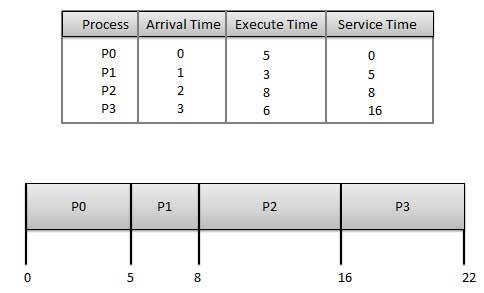# Operating System Scheduling algorithms

A Process Scheduler schedules different processes to be assigned to the CPU based on particular scheduling algorithms. There are six popular process scheduling algorithms which we are going to discuss in this chapter −

• First-Come, First-Served (FCFS) Scheduling
• Shortest-Job-Next (SJN) Scheduling
• Priority Scheduling
• Shortest Remaining Time
• Round Robin(RR) Scheduling
• Multiple-Level Queues Scheduling

These algorithms are either non-preemptive or preemptive. Non-preemptive algorithms are designed so that once a process enters the running state, it cannot be preempted until it completes its allotted time, whereas the preemptive scheduling is based on priority where a scheduler may preempt a low priority running process anytime when a high priority process enters into a ready state.

## First Come First Serve (FCFS)

• Jobs are executed on first come, first serve basis.
• It is a non-preemptive, pre-emptive scheduling algorithm.
• Easy to understand and implement.
• Its implementation is based on FIFO queue.
• Poor in performance as average wait time is high.Wait time of each process is as follows −

Process Wait Time : Service Time - Arrival Time
P0 0 - 0 = 0
P1 5 - 1 = 4
P2 8 - 2 = 6
P3 16 - 3 = 13

Average Wait Time: (0+4+6+13) / 4 = 5.75

## Shortest Job Next (SJN)

• This is also known as shortest job first, or SJF

• This is a non-preemptive, pre-emptive scheduling algorithm.

• Best approach to minimize waiting time.

• Easy to implement in Batch systems where required CPU time is known in advance.

• Impossible to implement in interactive systems where required CPU time is not known.

• The processer should know in advance how much time process will take.

Given: Table of processes, and their Arrival time, Execution time

Process Arrival Time Execution Time Service Time
P0 0 5 0
P1 1 3 5
P2 2 8 14
P3 3 6 8Waiting time of each process is as follows −

Process Waiting Time
P0 0 - 0 = 0
P1 5 - 1 = 4
P2 14 - 2 = 12
P3 8 - 3 = 5

Average Wait Time: (0 + 4 + 12 + 5)/4 = 21 / 4 = 5.25

## Priority Based Scheduling

• Priority scheduling is a non-preemptive algorithm and one of the most common scheduling algorithms in batch systems.

• Each process is assigned a priority. Process with highest priority is to be executed first and so on.

• Processes with same priority are executed on first come first served basis.

• Priority can be decided based on memory requirements, time requirements or any other resource requirement.

Given: Table of processes, and their Arrival time, Execution time, and priority. Here we are considering 1 is the lowest priority.

Process Arrival Time Execution Time Priority Service Time
P0 0 5 1 0
P1 1 3 2 11
P2 2 8 1 14
P3 3 6 3 5Waiting time of each process is as follows −

Process Waiting Time
P0 0 - 0 = 0
P1 11 - 1 = 10
P2 14 - 2 = 12
P3 5 - 3 = 2

Average Wait Time: (0 + 10 + 12 + 2)/4 = 24 / 4 = 6

## Shortest Remaining Time

• Shortest remaining time (SRT) is the preemptive version of the SJN algorithm.

• The processor is allocated to the job closest to completion but it can be preempted by a newer ready job with shorter time to completion.

• Impossible to implement in interactive systems where required CPU time is not known.

• It is often used in batch environments where short jobs need to give preference.

## Round Robin Scheduling

• Round Robin is the preemptive process scheduling algorithm.

• Each process is provided a fix time to execute, it is called a quantum.

• Once a process is executed for a given time period, it is preempted and other process executes for a given time period.

• Context switching is used to save states of preempted processes.Wait time of each process is as follows −

Process Wait Time : Service Time - Arrival Time
P0 (0 - 0) + (12 - 3) = 9
P1 (3 - 1) = 2
P2 (6 - 2) + (14 - 9) + (20 - 17) = 12
P3 (9 - 3) + (17 - 12) = 11

Average Wait Time: (9+2+12+11) / 4 = 8.5

## Multiple-Level Queues Scheduling

Multiple-level queues are not an independent scheduling algorithm. They make use of other existing algorithms to group and schedule jobs with common characteristics.

• Multiple queues are maintained for processes with common characteristics.
• Each queue can have its own scheduling algorithms.
• Priorities are assigned to each queue.

For example, CPU-bound jobs can be scheduled in one queue and all I/O-bound jobs in another queue. The Process Scheduler then alternately selects jobs from each queue and assigns them to the CPU based on the algorithm assigned to the queue.